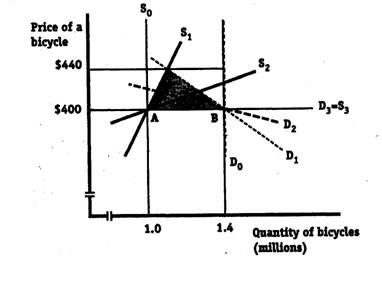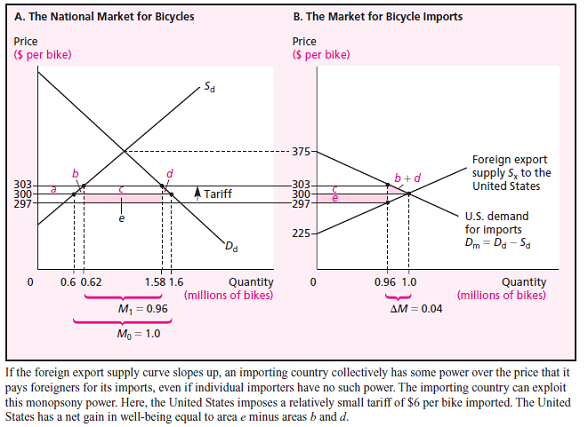# International Economics Study Set 9

## Quiz 7 : Analysis of a TariffLooking for Economics Homework Help?

## Quiz 7 :Analysis of a Tariff

Question Type"A tariff on imports of a product hurts domestic consumers of this product more than it benefits domestic producers of the product." Do you agree or disagree Why
Free
Essay

A tariff is an import duty or tax that is imposed on the imported goods in order to give protection to the domestic producer of those imported goods. Moreover, a tariff earns government some amount of revenue. Higher the amount of tariff, higher will be protection. The amount of tariff that wipes out all the imports is called a prohibitive tariff.
A country will take part in trade when it finds some prices lower that the domestic equilibrium price. But as a result domestic producers get affected. The producer surplus due to imports reduces and consumer surplus increases abruptly. A government imposes tariff, the world price becomes higher by the amount of tariff. As a result, now while importing consumers have to pay much higher than before to import and even higher to obtain the same domestically. However, producers receives a much higher price and there by revenue due to imposition of tariff domestically.
Hence, it can be said that a tariff hurts a consumer more than it benefits the domestic producers.

TagsWhat is the consumption effect of a tariff How would you describe it in words, without reference to any diagram or numbers How would you show it on a diagram, and how would you compute its value
Free
Essay

The consumption effect of a tariff is the loss of consumer surplus for the units that consumers would consume with free trade but do not consume when the tariff increases the domestic price. The tariff "artificially" raises the domestic price and causes some consumers to buy less of the product. On a diagram like Figure 8.3 or 8.4A, it is the triangular area d.

TagsSuppose that Canada produceS1.0 million bicycles a year and imports another 0.4 million; there is no tariff or other import barrier. Bicycles sell for $400 each. Parliament is considering a$40 tariff on bicycles like the one portrayed in Figures 8.2 through 8.4. What is the maximum net national loss that this could cause Canada What is the minimum national loss if Canada is a small country that cannot affect the world price ( Hint: Draw a diagram like Figure 8.4 and put the numbers given here on it. Next, imagine the possible positions and slopes of the relevant curves.)
Free
Essay

For a small country the world price of $400 will not be affected by the tariff. The size of the net national loss from imposing a$40 tariff will depend on the shapes of the domestic supply and demand curves. The graph shows several possible domestic supply and demand curves. (We will assume that supply and demand are straight lines.)
The maximum net national loss occurs when the two triangles of deadweight loss are as large as possible. The maximum loss occurs when the $40 tariff just eliminates all imports, so that the country shifts to no trade with a domestic price of$440. The tariff imposes a full $40 price distortion on the full amount of free-trade imports of 0.4 million per year. In the graph, any curves like S1 and D1 , which have free-trade at A and B and intersect each other at a price of$440, will cause a net national loss shown by the shaded triangle. The size of the loss is (½)•(1.4 - 1.0)•($40) =$8 million.
The minimum net national loss is zero. This can occur in any of several extreme cases. First, if both the supply curve and the demand curve are vertical (S0 and D0 ), then the domestic price increases to $440, but there are no changes of quantities. With no distortion of domestic producer and consumer decisions, there are no triangles of inefficiency. Second, if the domestic demand curve is flat (D 3 ), then domestic consumers receive no consumer surplus at the price of$400, and they will not pay more than $400 per bike. When the tariff is imposed, imports fall to zero, domestic price remains at$400, and domestic production remains aT1.0 million bikes. There are no triangles of loss. Third, if the domestic supply curve is flat (S 3 ), then domestic producers receive no producer surplus at the price of $400, and they can supply more bikes at the same price. When the tariff is imposed, imports fall to zero, domestic price remains at$400, and domestic production increases to match domestic consumption aT1.4 million bikes. There are no triangles of loss.TagsFor the international trade market for bicycles shown in Figure 8.5, demonstrate that a rather large tariff, for instance, a tariff which resulted in imports of 0.33 million bicycles, would not be an optimal tariff for the importing country.FIGURE 8.5 A Large Country Imposes a Small Tariff
Essay